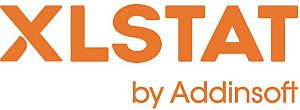# XLSTAT - Histograms

## What is a histogram

The histogram is one of the most frequently used display tools as it gives a very quick idea of the distribution of a sample of continuous or discrete data.

XLSTAT offers several options to create the histogram that will suit better your data:

## Options for plotting a histogram in XLSTAT

### Intervals definition

To make it easier to obtain histograms, XLSTAT lets you create histograms either by defining the number of intervals, their width or by specifying the intervals yourself. The intervals are considered as closed for the lower bound and open for the upper bound.

### Cumulative histogram

Create cumulative histograms either by cumulating the values of the histogram or by using the empirical cumulative distribution.

### Comparison to a theoretical distribution

XLSTAT lets you compare the histogram with a theoretical distribution whose parameters have been set by you. However, if you want to check if a sample follows a given distribution, you can use the distribution fitting tool to estimate the parameters of the distribution and if necessary check if the hypothesis is acceptable.

XLSTAT provides the following distributions:

• Arcsine
• Bernoulli
• Beta (2 options)
• Binomial
• Negative binomial type I and II
• Chi-square
• Erlang
• Exponential
• Fisher
• Fisher-Tippett
• Gamma
• GEV (Generalized Extreme Values)
• Gumbel
• Logistic
• Lognormal
• Normal
• Standard normal
• Pareto
• PERT
• Poisson
• Student
• Trapezoidal
• Uniform and Uniform discrete
• Weibull (3 options)This analysis is available in the XLStat-Basic addin for Microsoft ExcelKovach Computing Services (KCS) was founded in 1993 by Dr. Warren Kovach. The company specializes in the development and marketing of inexpensive and easy-to-use statistical software for scientists, as well as in data analysis consulting.

### Get in Touch

• Email:
sales@kovcomp.com